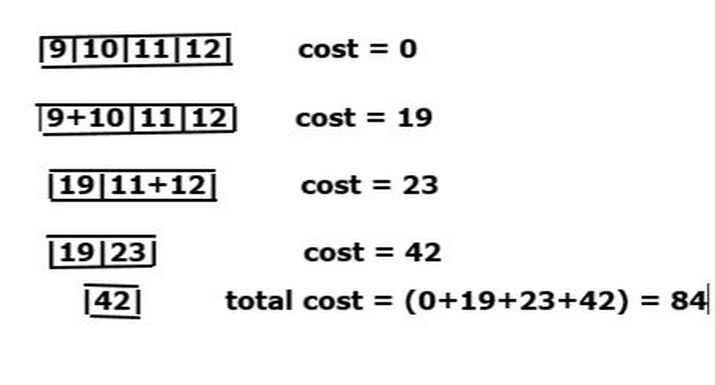# Minimum cost of reducing Array by merging any adjacent element repetitively

In C++ we have a pop() function to remove the element from the beginning. The top() function returns the reference of the first element of the priority_queue whereas the push() function is used to insert an element on it. A priority queue is a part of the data structure that manages elements based on their values.

In this article, we will learn the minimum cost of reducing an array by merging any adjacent element repetitively.

We will draw the array of size 4 and add the adjacent element repetitively.## Syntax

The following syntax used in the program −

priority_queue< int, vector<int>, greater<int> >name_of_queue;


### Explanation

This is the syntax of the min heap of the priority queue by default c++ creates the max heap of the priority queue.

int − This is the STL container that collects the object of the same type.

greater<int> - This is a comparator class used to compare an object of user-defined classes.

## Algorithm

• We will start the program with header files namely ‘iostream’, ‘queue’, and ‘vector’.

• Following that we are defining a global function named ‘findMinimumCost’ which accepts a two-parameter of integer value i.e. ‘arr[]’ and ‘n’ to verify it for minimum cost to reduce the array by merging any adjacent element.

• Then we start initializing the variable cost to ‘0’ which will later add to the ‘cost’ variable and define priority queue ‘ab’ to store the elements of an array.

• We are using for loop to traverse the input array and add each element to the priority queue ‘ab’.

• Then we will create a while loop to check the condition of priority queue ‘ab’. If it has more than 1 element then it will extract the two smallest elements from the queue.

• After extracting the elements by using top() and pop() predefined functions, we will add the sum to the cost and insert the sum into a queue which will reduce the minimum cost by merging the adjacent element.

• After processing all the conditions, the function returns the resultant value of ‘cost’.

• Now we will start the main function and store the value ‘4’ to ‘n’ variable which represents the size of the variable. Next, store the array elements in the ‘arr[]’ variable.

• We will call the ‘findMinimumCost()’ function that accepts two parameters ‘arr’ and ‘n’ to pass the input and this function call is stored to the variable ‘minimumCost’.

• Finally, we print the statement “Minimum cost of reducing the array:” with help of the ‘minimumCost’ variable.

## Example

In this program, we are going to the minimum cost of reducing the array by merging any adjacent element repetitively.

#include <iostream>
#include <queue>
#include <vector>

using namespace std;
int findMinimumCost(int arr[], int n) {
int cost = 0;
priority_queue<int, vector<int>, greater<int>> ab;

// add elements of the array to the priority queue
for (int i = 0; i < n; i++) {
ab.push(arr[i]);
}

// reducing the array by merging adjacent elements
while (ab.size() > 1) {
int x = ab.top();
ab.pop();
int y = ab.top();
ab.pop();
int sum = x + y;
cost += sum;
ab.push(sum);
}
return cost;
}

int main() {
int n = 4;
// size of array
int arr[] = {9, 10, 11, 12};
int minimumCost = findMinimumCost(arr, n);
cout << "Minimum cost of reducing the array: " << minimumCost << endl;
return 0;
}


## Output

Minimum cost of reducing the array: 84


## Conclusion

We explored the concept of minimum cost of reducing the array by merging any adjacent element repetitively. We saw the difference between pop() and top() in this program. This technique is mostly used in applications such as load balancing, priority scheduling in OS, interrupt handling, and data compression.

Updated on: 10-May-2023

164 Views